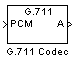# G711 Codec

Quantize narrowband speech input signals

## Library

Quantizers

`dspquant2`

•## Description

The G711 Codec block is a logarithmic scalar quantizer designed for narrowband speech. Narrowband speech is defined as a voice signal with an analog bandwidth of 4 kHz and a Nyquist sampling frequency of 8 kHz. The block quantizes a narrowband speech input signal so that it can be transmitted using only 8-bits. The G711 Codec block has three modes of operation: encoding, decoding, and conversion. You can choose the block's mode of operation by setting the Mode parameter.

If, for the Mode parameter, you choose ```Encode PCM to A-law```, the block assumes that the linear PCM input signal has a dynamic range of 13 bits. Because the block always operates in saturation mode, it assigns any input value above ${2}^{12}-1$ to ${2}^{12}-1$ and any input value below $-{2}^{12}$ to $-{2}^{12}$. The block implements an A-law quantizer on the input signal and outputs A-law index values. When you choose ```Encode PCM to mu-law```, the block assumes that the linear PCM input signal has a dynamic range of 14 bits. Because the block always operates in saturation mode, it assigns any input value above ${2}^{13}-1$ to ${2}^{13}-1$ and any input value below $-{2}^{13}$ to $-{2}^{13}$. The block implements a mu-law quantizer on the input signal and outputs mu-law index values.

If, for the Mode parameter, you choose ```Decode A-law to PCM```, the block decodes the input A-law index values into quantized output values using an A-law lookup table. When you choose ```Decode mu-law to PCM```, the block decodes the input mu-law index values into quantized output values using a mu-law lookup table.

If, for the Mode parameter, you choose ```Convert A-law to mu-law```, the block converts the input A-law index values to mu-law index values. When you choose `Convert mu-law to A-law`, the block converts the input mu-law index values to A-law index values.

Note

Set the Mode parameter to ```Convert A-law to mu-law``` or `Convert mu-law to A-law` only when the input to the block is A-law or mu-law index values.

If, for the Mode parameter, you choose ```Encode PCM to A-law``` or `Encode PCM to mu-law`, the Overflow diagnostic parameter appears on the block parameters dialog box. Use this parameter to determine the behavior of the block when overflow occurs. The following options are available:

• `Ignore` — Proceed with the computation and do not issue a warning message.

• `Warning` — Display a warning message in the MATLAB® Command Window, and continue the simulation.

• `Error` — Display an error dialog box and terminate the simulation.

Note

Like all diagnostic parameters on the Configuration Parameters dialog box, Overflow diagnostic parameter is set to `Ignore` in the code generated for this block by Simulink® Coder™ code generation software.

## Parameters

Mode
• When you choose `Encode PCM to A-law`, the block implements an A-law encoder.

• When you choose `Encode PCM to mu-law`, the block implements a mu-law encoder.

• When you choose `Decode A-law to PCM`, the block decodes the input index values into quantized output values using an A-law lookup table.

• When you choose `Decode mu-law to PCM`, the block decodes the input index values into quantized output values using a mu-law lookup table.

• When you choose ```Convert A-law to mu-law```, the block converts the input A-law index values to mu-law index values.

• When you choose ```Convert mu-law to A-law```, the block converts the input mu-law index values to A-law index values.

Overflow diagnostic

Use this parameter to determine the behavior of the block when overflow occurs.

• Select `Ignore` to proceed with the computation without a warning message.

• Select `Warning` to display a warning message in the MATLAB Command Window and continue the simulation.

• Select `Error` to display an error dialog box and terminate the simulation.

This parameter is only visible if, for the Mode parameter, you select `Encode PCM to A-law` or `Encode PCM to mu-law`.

## References

ITU-T Recommendation G.711, “Pulse Code Modulation (PCM) of Voice Frequencies,” General Aspects of Digital Transmission Systems; Terminal Equipments, International Telecommunication Union (ITU), 1993.

## Supported Data Types

PortSupported Data Types

PCM

• 16-bit signed integers

A

• 8-bit unsigned integers

mu

• 8-bit unsigned integers